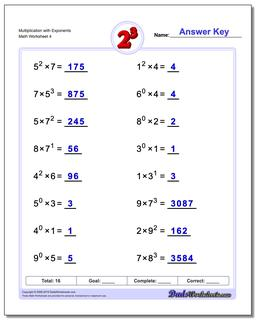# Math Worksheets: Exponents: Exponents: Multiplication with Exponents (Fourth Worksheet)## Multiplication with Exponents (Fourth Worksheet)

PropertyValue
DescriptionMultiplication with Exponents: Practice exponents worksheets mixing exponents with simple multiplication. Again, these are fantastic exercises for enhancing student comprehension of basic order of operations concepts where exponents are involved. (Fourth Worksheet)
Resource TypeWorksheet